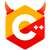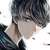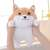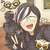# 3342. 经典的猜数游戏#include <iostream>
#include <string>
using namespace std;

int main() {
int bg = -1'000'000'000;
int ed = 1'000'000'001;
while (true) {
int mid = bg + (ed - bg) / 2;
cout << mid << endl;
string a;
cin >> a;
if (a == "big")
ed = mid;
else if (a == "small")
bg = mid + 1;
else
break;
}
}#include <iostream>

using namespace std;

int main() {
int l = -1e9, r = 1e9;
while (l < r) {
int mid = l + r >> 1;
cout << mid << endl; // 交互题。先猜，把mid输出到标准输出流

string s;
cin >> s; // 对于我的回答，你要从标准输入流中读入
if (s == "equal") break;

if (s == "big") r = mid;
else l = mid + 1;

}

return 0;
}#include<vector>
#include<iostream>
#include<queue>
#include<algorithm>
#include<string>

using namespace std;

int main()
{
long lmax=1000000001;
long lmin=-1000000001;
long res;

for(int i=0;i<32;i++){
long res=(lmax+lmin)/2L;
cout<<res<<endl;
cout.flush();
lmax=res;
}
lmin=res;
}
break;
}
}
}C++ ostream 的 endl 本身就会刷新缓冲区，没有必要显式地调用 os.flush()#include <iostream>
#include <string>
using namespace std;
int main()
{
int max = 1e9;
int min = -1e9;
int mid = 0;
cout << mid << endl;
string input;
while (cin >> input && input != "equal")
{
if (input == "big")
max = mid - 1;
else
min = mid + 1;
mid = (max + min) / 2;
cout << mid << endl;
}
}